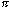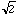# Difference Between Rational and Irrational Numbers

What is the difference Between Rational and Irrational Numbers?

Rational Numbers: – Every integer and fractions are rational numbers. It can always be denoted as p/q, where p and q are integers and q is not equal to 0.

Thus for example the rationals include {0, 5/2, -18, -4/3, 27/5}. We unually write rational numbers in their lowest terms, for example 8/10 is usually written 4/5. We commonly write rationals in decimal form, so that 1/4 is the same as 0.25, 13/8 = 1.625 and 4/5 = 0.8. Some rationals, however, when written in decimal form don’t stop a few places after the decimal. For example 1/3 = 0.333…, 10/11 = 0.909090… and 3/13 = 0.230769230769… When you write a rational number in decimal form you obtain either a decimal that stops after a finite number of terms, or a pattern that repeats as in the latter three examples.

Irrational Numbers: – Infinite non-recurring decimal number can be expressed as an irrational number.

If you construct a decimal that does not terminate and does not repeat it is not a rational number. For example 0.102003000400005… Numbers that are not rational are called irrational. The most famous of these is the ratio of the circumference of a circle to its diameter, called.is approximately 3.14159265358979323846; no matter how far we take the decimal expansion, it never repeats. Another irrational number iswhich is approximately 1.4142135623731.#### Vineet Patawari

Hi, I'm Vineet Patawari. I fell in love with numbers after being scared of them for quite some time. Now, I'm here to make you feel comfortable with numbers and help you get rid of Math Phobia!

## 2 thoughts to “Difference Between Rational and Irrational Numbers”

1.Mohan says:

Hi ,
This is mohan. Since I am not from science or maths background it’s very hard for me to understand this Rational or Irrational concept. Plz help me to understand this concept indepth with some good examples..

2.NANDEESH H N says: